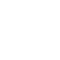# Active Tuning of Spring Constant and Resonant Frequency

Mechanical characteristics such as spring constants and resonant frequencies of beams and membranes can be changed by introducing strain. In this section, we focus on discussing methods for tuning characteristics of cantilever beams to exemplify the methodology.

For cantilevers, longitudinal strain can be introduced by axial or transverse loading forces. We will discuss these two cases separately below.

First, an axial tension can alter the spring constant and resonant frequency. This is analogous to a violinist tuning the sound of a string by adjusting its tension.

The longitudinal tuning force can be introduced by lateral electrostatic force, or thermal expansion. (In the same token, the shift of resonance frequency can be used to characterize intrinsic stress of fixed-fixed beams .) The resonant frequency of a cantilever under a longitudinal strain εs is

$w=w_{0} \sqrt{1+\frac{2 L^{2}}{7 h^{2}} \varepsilon_{s}}$

where w0, h and L are the untuned resonant frequency, and the thickness and length of the beam.

The force constant and resonance frequency of a cantilever can be tuned by transverse forces. To illustrate this case, think about a child standing at the end of a diving board and bouncing lightly. Let us assume that the board dips below the horizontal level. The child feels the board has a certain mechanical stiffness.

Now, imaging an adult pulling down at the same end of the diving board. The child will feel that the diving board becomes softer (more displacement under a given weight) when there is an active pulling force.

In other words, the diving board appears to be softer to the child. Beam softening results whenever the bias force point in the opposite direction of the cantilever restoring force.

The apparent force constant changes from k to keff. The transverse loading force may be applied electrostatically [69, 70], thermally , or magnetically.